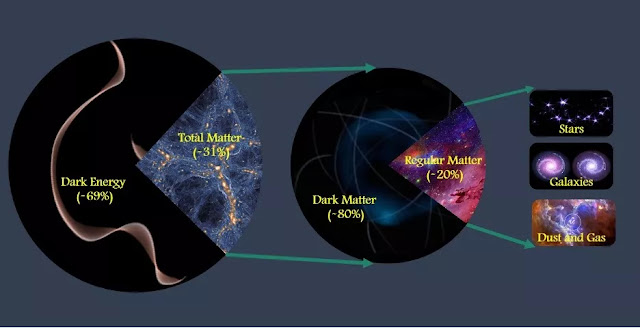### Six Protons per Cubic Meter

"I have deep faith that the principle of the Universe will be beautiful and simple- Albert Einstein
_______________________________________________________________

Of all the space-related scientific fields I try to cover on Astronomical Returns, by far the most challenging to write about is theoretical physics (unsurprisingly). But last month I saw a study get published about the matter density of the entire Universe that I found really fascinating, not just for the mathematical enormity of the task, but also for its significant implications in the field of cosmology! Here's the deal:

Cosmologists have long sought a definitive answer to the question, "What is the ultimate fate of the Universe?" Observations performed by Edwin Hubble in the 1920s unequivocally demonstrated that the Universe is currently expanding in all directions, as evidenced by the apparent redshift in the light emitted by distant galaxies. It's this groundbreaking discovery that forms the basis of the Big Bang theory. Naturally, the question that arose in the subsequent decades is whether or not the Universe will continue to expand forever, or if at some point the collective gravity of the Universe will be enough to reverse the expansion. The answer depends on the matter density of the entire Universe (denoted by the variable $\Omega$), and it boils down to three broad possibilities:

1. Closed universe ($\Omega > 1$): There is enough mass (i.e. gravity) to eventually reverse the expansion of the Universe. The Universe will end in a Big Crunch, the opposite of a Big Bang whereby all matter recollapses into a single point
2. Open universe ($\Omega < 1$): There is not enough mass to halt the cosmological expansion. The Universe will expand forever, leaving nothing but a frozen void after all matter has decayed
3. Flat universe ($\Omega = 1$): There is some critical matter density where expansion continues forever, but at increasingly slower rates until the rate of expansion levels out entirely. The end result is similar to the Open universeHere's a great diagram of the three scenarios (Closed, Flat, and Open from left to right) | Credit: here

This concept of the density parameter $\Omega$ is given by the expression $\rho / \rho_c$, where $\rho_c$ is the critical matter density needed to maintain a flat Universe and $\rho$ is whatever the matter density of the Universe actually is (don't be afraid of all the variables, it's just a simple ratio!). Amazingly, we can calculate the value of $\rho_c$, it's given by the equation $\rho_c = \frac{3H^2}{8 \pi G}$ (the derivation of this comes from the Friedmann equations, which is far beyond my scope of knowledge). $H$ is the Hubble constant, which is the observed rate of expansion of the Universe, and $G$ is the universal gravitational constant

Knowing that $H = 67.4 \thinspace km/s/Mpc$ and $G = 6.67 * 10^{-11}\thinspace m^3 kg^{-1} s^{-2}$,

We find that $\rho_c = 8.5 * 10^{-27} \thinspace kg/m^3$

This corresponds to a matter density of about 6 protons per cubic meter as the critical matter density for a flat UniverseEdwin Hubble is credited with discovering the expansion of the Universe. Now you know the namesake of the Hubble Space Telescope!

Great, so now if we can just calculate the actual matter density of the Universe, $\rho$, we can compare to $\rho_c$ and figure out what kind of Universe we live in. That's where this new study comes in, as although similar studies have been performed in the past, this latest census is the most comprehensive. The team at UC Riverside analyzed the prevalence and motions of galactic superclusters, a reliable metric for estimating the total matter in the Universe. Present-day galaxy clusters formed after billions of years of matter aggregating and collapsing under their own collective gravity; such accumulation would have been very sensitive to early cosmological conditions, namely the prevalence of matter. So the team of astronomers first performed numerous observations to create a catalog of galaxy clusters, then ran multiple simulations to determine what sort of matter density would yield the dispersion of matter we see today"Like Goldilocks, the team compared the number of galaxy clusters they measured with predictions from numerical simulations to determine which answer was 'just right'." | Credit: UCR

Woaaaahh, what's going on? Am I the only one amazed by the fact that the cosmos happens to have just the right amount of matter to make it a flat Universe, where $\rho = \rho_c$? And yet, that's exactly what the data suggests, and is in line with past observations that have mostly led the scientific community to believe we indeed live in a flat Universe. Perhaps it's an underlying requirement for the Universe to even exist in the stable form we know of, or maybe it's just an enormous cosmic coincidence. But for whatever reason, cosmologists have calculated the value of $\Omega$ to be equal to 1, within a 0.4% margin of errorWe really are just a tiny slice of the cosmic pie

Moreover, the study by UCR is also in close alignment with prior observations of another calculation: the ratio of dark matter and dark energy. Dark energy (the yet-unknown repulsive force behind the accelerating expansion of the Universe) is known to be 69% of the total energy-matter content of the Universe, and of the remaining 31% that's matter, 80% of it is dark matter (the yet-undiscovered mass content that holds rotating galactic clusters together). So while the total matter dispersion of the Universe may be six protons per cubic meter, only a fraction of it is the regular matter we're familiar with and that makes up all the planets, stars, and galaxies. Whatever else is out there, we simply don't knowFrom Calvin and Hobbes, my all-time favorite comic!

To learn more about the matter density of the Universe and the great cosmic coincidence we happen to find ourselves in, this video is fantastic